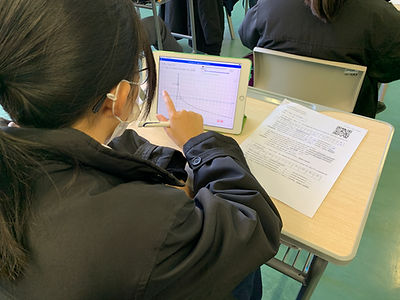top of page## Mathematics

• Aims

• develop the ability to think critically and creatively, to conceptualize, inquire and reason mathematically, and to use mathematics to formulate and solve problems in daily life as well as in mathematical contexts and other disciplines;

• develop the ability to communicate with others, express their views clearly and logically in mathematical language;

• develop the ability to manipulate numbers, symbols and other mathematical objects;

• develop number sense, symbol sense, spatial sense, measurement sense and the capacity to appreciate structures and patterns; and

• develop a positive attitude towards mathematics learning and an appreciation of the aesthetic nature and cultural aspect of mathematics.

• Curriculum Framework

• Strands of KS3 (S1 - S3)

• Number and Algebra

• Measures, Shape and Space

• Data Handling

• Strands of KS4 (S4 - S6) Compulsory Part

• Number and Algebra

• Measures, Shape and Space

• Data Handling

• Areas of KS4 (S4 - S6) Extended Part

• Foundation knowledge​

• Algebra

• Calculus

• Statistics

• Learning Strategies

• Clear lesson goals​

• Show and tell

• Questioning to check for understanding

• Summaries new learning in a graphical way

• Plenty of practice

• Provide students with feedback

• Be flexible about how long it takes to learn

• Get students working together

• Teach strategies not just content

• Nurture meta-cognition

• Assessment

A. Public examination (HKDSE)​

• Compulsory Part

• Extended Part

B. Internal Assessment​bottom of page